# Ray Optics and Optical Instrument - Critical Angle and Total Internal Reflection

Critical Angle: When the ray of light goes from denser to rare medium, it bends away from the normal and as the angle of incidence in denser medium increases, the angle of refraction in rare medium also increases at a certain angle, then angle of refraction becomes 90°. This angle of incidence is called Critical Angle.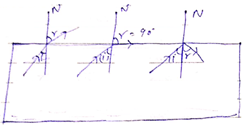Formula, according to snell’s law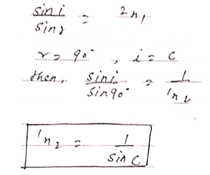Total Internal Reflections: When angle of incidence exceeds the critical angle then light may come back into the same medium after reflection from interface. This phenomenon is called Total Internal Reflection.

Application of Total Internal reflection:

1. Mirage: There is the optical illusion in hot places or at cool solid road in summer days. This is due to total internal reflection. In this process the inverted image is produced, so a possibility of water is inverted but there is no such place of water.

The inverted image is formed due to the total internal reflection.

Reflection of the space above the earth is divided into the layers. The layers near to the earth surface are rare and optical density increase upward.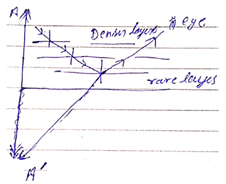Hence, the ray of the light starts from point A of the tree goes several refractions from denser to rare and its incident angle increases regularly. At a particular incident angle becomes more than critical angle, the ray goes back external reflection and its image is seen by the observer at point A.

2. Brillians of Diamond: The refractive index of diamond 2.42

Hence the critical angle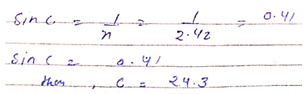Due to small critical angle and the shape made by cutting, so that incident angle is always greater than Critical angle hence due to multiple total internal reflection, the transmitted light is more bright.

3. Optical Fibre: It has three parts.

1. Core
3. Cover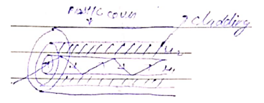Core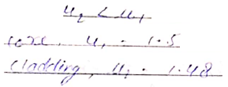Construction: The centre wire is made of glass or quartz through which optical rays passes its refractive index. About 1-5 another material surrounding it is called Cladding. Its refractive index is less then the refractive index of the core. It is around 1.48. The whole combination is provided by plastic cover.

Working: When the signal of optical waves incident on an angle greater than critical angle, it gets total internal reflection. At the interface of core and cladding this way optical waves is transmitted from one place to another places.

Application:

1. Communication
2. Internet
3. Lightning & decoration

4. Totally Reflection Prism: Totally reflection prism is isosceles prism which one angle 90° and other at 45° each.

Working: There are two types of internal reflection, 90 and 180 degrees. The refractive index of prism glass material is 1.5 and its critical angle 41.8°.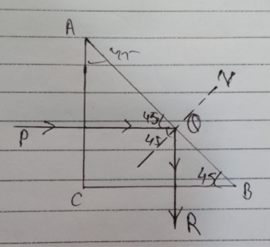1. Deviation 90°: When the ray of light PQ falls perpendicular to the surface AC, it passes un deviated from the surface and falls at an angle 45°. On AB surface since incident angle is more than critical angle hence it gets total internal reflection and goes to the path QK. That the incident ray gets deviation by angle 90°
2. Deviation 180°: Here ray deviation two times by 90° so it is called 180° deviations.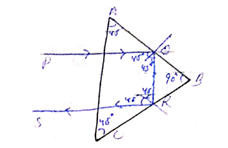Post By : Nek Singh Yadav 02 May, 2020 435 views Physics